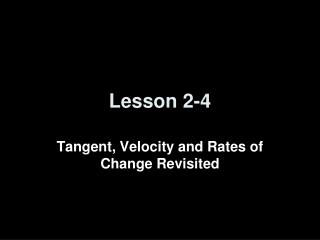DownloadDownload PresentationLesson 2-4

# Lesson 2-4

Télécharger la présentation## Lesson 2-4

- - - - - - - - - - - - - - - - - - - - - - - - - - - E N D - - - - - - - - - - - - - - - - - - - - - - - - - - -
##### Presentation Transcript

1. Lesson 2-4 Tangent, Velocity and Rates of Change Revisited

2. Objectives • Identify the average and instantaneous rates of change

3. Vocabulary • Average rate of change – ∆y/∆x (the slope of the secant line between two points on the curve) • Instantaneous rate of change – lim ∆y/∆x (∆x→0) (the slope of the tangent line at a point on the curve)

4. Average Rate of Change Average Rate of Change – slope of the secant line Q f(a+h) P f(a) secant h = b - a a h b ∆y f(a+h) – f(a) ms = ----- = ----------------- ∆x h

5. Instantaneous Rate of Change Instantaneous Rate of Change– slope of the tangent line (derivative of the function evaluated at the point) mt ms Q f(a+h) P As Q gets closer and closer to P, h (∆x) gets closer to 0; and the slope of the secant line, ms, approaches the slope of the tangent line, mt f(a) a h b ∆y f(a+h) – f(a) mt = lim ----- = lim ----------------- ∆x h h→0 ∆x→0

6. Average vs Instantaneous Rate of Change Average Rate of Change – slope of the secant line Q f(a+h) ∆y f(a+h) – f(a) ms = ----- = ----------------- ∆x h P f(a) a h b Instantaneous Rate of Change – slope of the tangent line (derivative of the function evaluated at the point) mt Q As Q gets closer and closer to P, h (∆x) gets closer to 0; and the slope of the secant line, ms, approaches the slope of the tangent line, mt f(a+h) P f(a) ∆y f(a+h) – f(a) mt = lim ----- = lim ----------------- ∆x h h→0 ∆x→0 a h b

7. Example 1 Find the slope of the tangent line to the curve f(x) = 3 – 2x – 2x2 where x = 1. f(1+h) – f(1) lim --------------------- = h0 h f(a+h) – f(a) lim --------------------- = h0 h (-1 - 6h - 2h²) – (-1) lim --------------------------- = h0 h f(1) = 3 - 2 - 2 = -1 f(1+h) = 3 - 2(1+h) - 2(1+h)² = 3 - 2 - 2h - 2 - 4h -2h² = -1 - 6h - 2h² -6h -2h² lim ------------- = h0 h lim -6 – 2h = -6 h0

8. Example 2 Find the slope of the tangent line (using the definition) where f(x) = 1/(x-1) and a = 2. f(2+h) – f(2) lim --------------------- = h0 h f(a+h) – f(a) lim --------------------- = h0 h (1/(h+1)) – (1) lim --------------------- = h0 h f(2) = 1/(2-1) = 1 f(2+h) = 1/(2+h – 1) = 1/(h + 1) - h / (h + 1) lim ---------------- = h0 h 1 h + 1 - h ------ - --------- = ----------- h + 1 h + 1 h + 1 - 1 lim ---------- = - 1 h0 h + 1

9. Example 3 Find the instantaneous velocity at time t = 3 seconds if the particle’s position at time is given by f(t) = t2 + 2t ft. f(3+h) – f(3) lim --------------------- = h0 h f(a+h) – f(a) lim --------------------- = h0 h (h² + 8h + 15) – (15) lim --------------------------- = h0 h f(3) = 9 + 6 = 15 f(3+h) = (3+h)² + 2(3+h) = h² + 6h + 9 + 6 + 2h = h² + 8h + 15 h² + 8h lim ------------- = h0 h lim h + 8 = 8 h0

10. Example 4 For a particle whose position at time t is f(t) = 6t2 - 4t +1ft.: a. Find the average velocity over the following intervals: • [1, 4] • [1, 2] • [1, 1.2] • [1, 1.01] ∆y Ave vel = ms = --------- ∆x f(4) – f(1) 81 – 3 78 ---------------- = ------------- = -------- = 26 4 – 1 3 3 f(2) – f(1) 17 - 3 ---------------- = ------------ = 14 2 – 1 1 f(1.2) – f(1) 4.84 - 3 1.84 ------------------ = -------------- = -------------- = 9.2 1.2 – 1 0.2 0.2 f(1.01) – f(1) 3.0806 - 3 .0806 -------------------- = --------------- = ------------- = 8.06 1.01 – 1 .01 .01

11. Example 4 continued For a particle whose position at time t is f(t) = 6t2 - 4t +1 ft.: b. Find the instantaneous velocity of the particle at t = 1 sec.   f(1+h) – f(1) lim --------------------- = h0 h f(a+h) – f(a) lim --------------------- = h0 h (6h² + 8h + 3) – (3) lim --------------------------- = h0 h f(1) = 6 - 4 + 1 = 3 f(1+h) = 6(1+h)² - 4(1+h) + 1 = 6h² + 12h + 6 – 4h – 4 + 1 = 6h² + 8h + 3 6h² + 8h lim ------------- = h0 h lim 6h + 8 = 8 h0

12. Summary • Summary: • Average rate of change is the slope of the secant line • Instantaneous rate of change is the slope of the tangent line • Limit of m secant = m tangent as ∆x →0